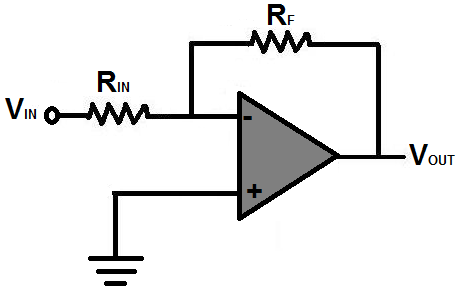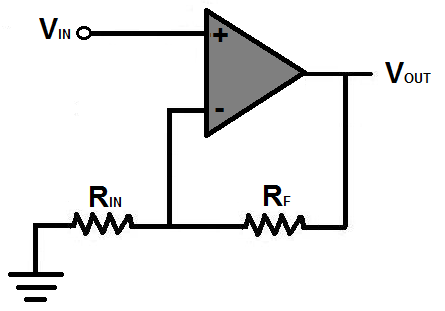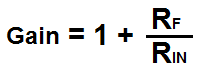﻿ Op Amp Gain Calculator ﻿# Op Amp Gain Calculator### Inverting Op Amp Gain CalculatorResistance, RIN: Ω (Ohms) KΩ (Kilohms) MΩ (Megohms) Resistance, RF: Ω (Ohms) KΩ (Kilohms) MΩ (Megohms)

0.00

This calculator calculates the gain of an inverting op amp based on the input resistor value, RIN, and the output resistor value, RF, according to the formula, Gain= RF/RIN.

To use this calculator, a user just inputs the value of resistor, RIN, and resistor, RF, and clicks the 'Submit' button and the answer is automatically computed and shown below. A user can specify each resistance value as either ohms (Ω), kilohms (KΩ), or megohms (MΩ). The output resultant value does not any units. It's a unitless result. It's simply the factor by which the input signal is multiplied by to produce the amplified output voltage.

The gain of an op amp signifies how much greater in magnitude the output voltage will be than the input. For example, an op amp with a resistor, RIN, of 1KΩ and a resistor, RF of 10KΩ, will have a gain of 10. This means that the output will be ten times greater in magnitude than the input voltage. So, for example, if the input voltage is 5V in magnitude, the output voltage will be 50V in magnitude.

Calculating the gain of an op amp is very important because many circuits such as audio amplifiers and volume control circuits need to utilize op amp gain in order to function correctly. Knowing the formulas for calculating the gain of op amps is crucial to having high-precision amplifier circuits.

### Noninverting Op Amp Gain CalculatorResistance, RIN: Ω (Ohms) KΩ (Kilohms) MΩ (Megohms) Resistance, RF: Ω (Ohms) KΩ (Kilohms) MΩ (Megohms)

0.00

This calculator calculates the gain of a noninverting op amp based on the input resistor value, RIN, and the output resistor value, RF, according to the formula, Gain= 1 + RF/RIN.

To use this calculator, a user just inputs the value of resistor, RIN, and resistor, RF, and clicks the 'Submit' button and the answer is automatically computed and shown below. A user can specify each resistance value as either ohms (Ω), kilohms (KΩ), or megohms (MΩ). The output resultant gain value does not have any units.

The gain of an op amp signifies how much greater in magnitude the output voltage will be than the input. For example, an op amp with a resistor, RIN, of 20KΩ and a resistor, RF of 100KΩ, will have a gain of 6. This means that the output will be 6 times greater in magnitude than the input voltage. So, for example, if the input voltage is 9V in magnitude, the output voltage will be 54V in magnitude.

Related Resources

﻿